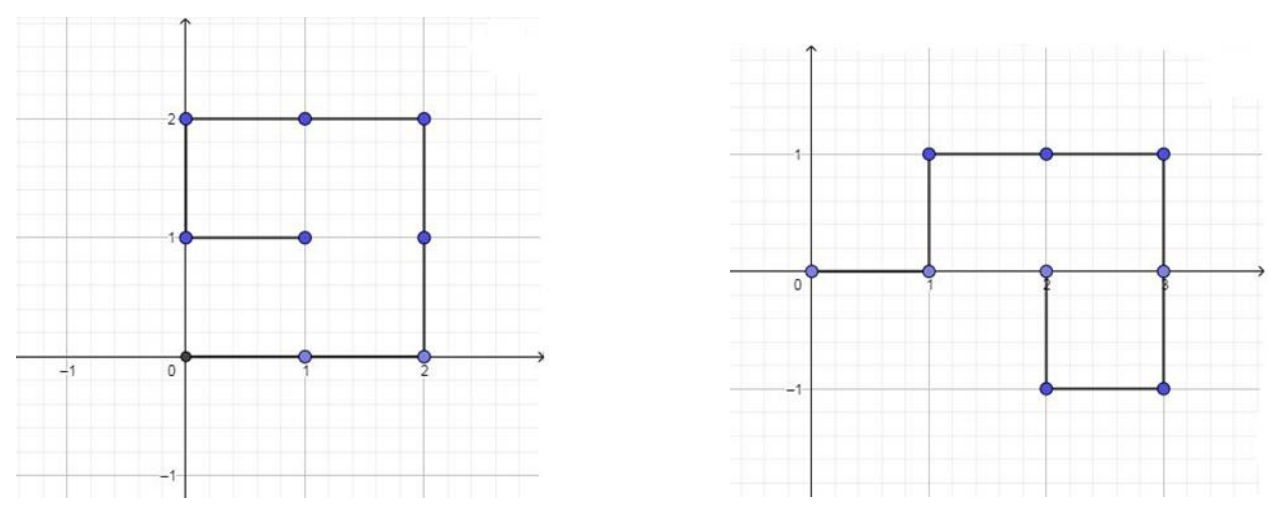시간 제한 메모리 제한 제출 정답 맞은 사람 정답 비율
2 초 512 MB 0 0 0 0.000%

## 문제

We form a sequence of points that are vertices with integer coordinates in a square grid. Each two consecutive points of the sequence define a single horizontal or vertical segment of length one. We call this sequence a walk. Consider such walks composed of n segments that are self-avoiding (i.e. segments in the walk are not intersecting themselves and do not touch each other, except any two consecutive segments). We also want the first segment in the walk to join the points with coordinates (0,0) and (1,0), and the first vertical segment to be going up.

Write program that computes the number of all self-avoiding walks on square grid that are trapped after n steps, i.e. which are not possible to continue, because adding the next (n + 1) segment will cause self-intersection.

An integer n.

## 출력

An integer equal to the requested number.

• 0 < n < 27

## 예제 입력 1

8


## 예제 출력 1

3


## 힌트

The two walks are (0,0) (1,0) (2,0) (2,1) (2,2) (1,2) (0,2) (0,1) (1,1) and (0,0) (1,0) (1,1) (2,1) (3,1) (3,0) (3, -1) (2, -1) (2,0), and they are depicted in the figures: